# EVEN and ODD

Use EVEN and ODD in Excel to round numbers. Use ISEVEN or ISODD in Excel to determine if a number is even or odd.

### Even

1. The EVEN function rounds a positive number up to the nearest even integer.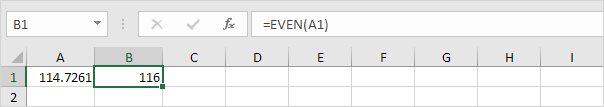2. The EVEN function rounds a negative number down to the nearest even integer.### Odd

1. The ODD function rounds a positive number up to the nearest odd integer.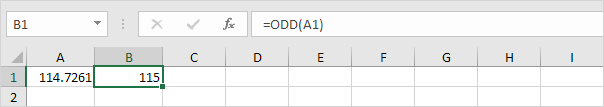2. The ODD function rounds a negative number down to the nearest odd integer.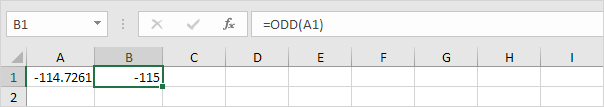### IsEven

The ISEVEN function returns TRUE if a number is even and FALSE if a number is odd.

1. The ISEVEN function below returns TRUE.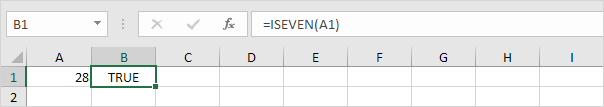2. The ISEVEN function below returns FALSE.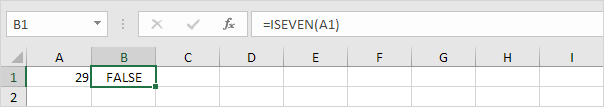Add the IF function to return Even or Odd.

3. The formula below returns Even.4. The formula below returns Odd.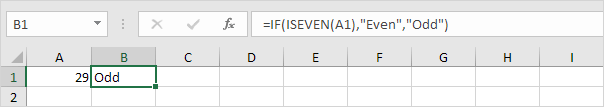### IsOdd

The ISODD function returns TRUE if a number is odd and FALSE if a number is even.

1. The ISODD function below returns TRUE.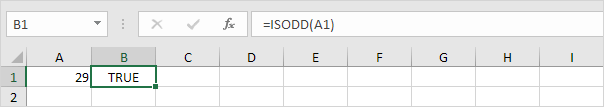2. The ISODD function below returns FALSE.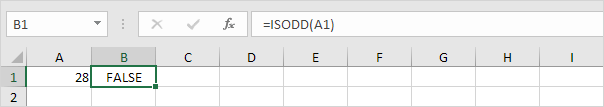3. Here's a cool example. Use conditional formatting and the ISODD function to highlight all odd numbers.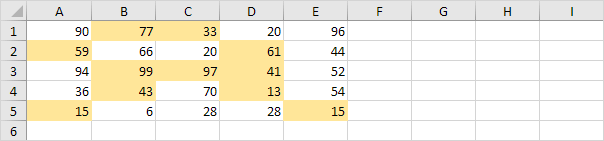Note: instead of using ISEVEN or ISODD to determine if a number is even or odd, use the MOD function in Excel.

Go to Next Chapter: Formula Errors